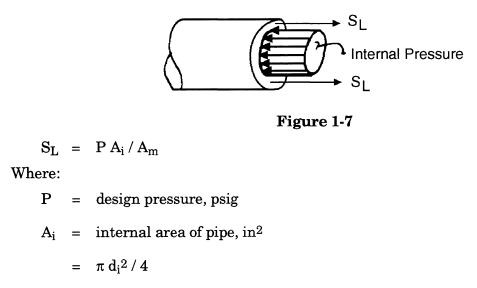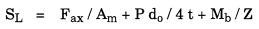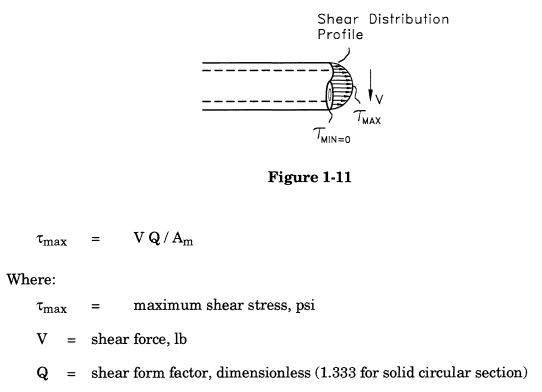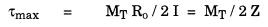Search

Normal stresses: Normal stresses are those acting in a direction normal to the face of the crystal structure of the material, and may be either tensile or compressive in nature. (In fact, normal stresses in piping tend more to tension due the predominant nature of internal pressure as a load case.) Normal stresses may be applied in more than one direction, and may develop from a number of different types of loads. For a piping system, these are discussed below:

Longitudinal stress: Longitudinal, or axial, stress is the normal stress acting parallel to the longitudinal axis of the pipe. This may be caused by an internal force acting axially within the pipe:A specific instance of longitudinal stress is that due to internal pressure:Replacing the terms for the internal and metal areas of the pipe, the previous equation may be written as:For convenience , the longitudinal pressure stress is often conservatively approximated as:Another component of axial normal stress is bending stress. Bending stress is zero at the neutral axis of the pipe and varies linearly across the cross-section from the maximum compressive outer fiber to the maximum tensile outer fiber. Calculating the stress as linearly proportional to the distance from the neutral axis:Maximum bending stress occurs where c is greatest — where it is equal to the outer radius:Summing all components of longitudinal normal stress:Hoop stress: There are other normal stresses present in the pipe, applied in directions orthogonal to the axial direction. One of these stresses, caused by internal pressure, is called hoop stress. This stress acts in a direction parallel to the pipe circumference.The magnitude of the hoop stress varies through the pipe wall and can be calculated by Lame's equation as:The hoop stress can be conservatively approximated for thin-wall cylinders, by assuming that the pressure force, applied over an arbitrary length of pipe, 1 (F = P di L), is resisted uniformly by the pipe wall over that same arbitrary length (Am = 2 tL), or:Radial stress: Radial stress is the third normal stress present in the pipe wall. It acts in the third orthogonal direction, parallel to the pipe radius. Radial stress, which is caused by internal pressure, varies between a stress equal to the internal pressure at the pipe's inner surface and a stress equal to atmospheric pressure at the pipe's external surface. Assuming that there is no external pressure, radial stress may be calculated as:Note that radial stress is zero at the outer radius of the pipe, where the bending stresses are maximized. For this reason, this stress component has traditionally been ignored during the stress calculations.

Shear stresses: Shear stresses are applied in a direction parallel to the face of the plane of the crystal structure of the material, and tend to cause adjacent planes of the crystal to slip against each other. Shear stresses may be caused by more than one type of applied load.

For example, shear stress may be caused by shear forces acting on the cross-section:These shear stresses are distributed such that they are maximum at the neutral axis of the pipe and zero at the maximum distance from the neutral axis. Since this is the opposite of the case with bending stresses, and since these stresses are usually small, shear stresses due to forces are traditionally neglected during pipe stress analysis.

Shear stresses may also be caused by torsional loads:Maximum torsional stress occurs where c is maximized — at the outer radius:Summing the individual components of the shear stress, the maximum shear stress acting on the pipe cross-section is:Example Stress Calculations:

As noted above, a number of the stress components described above have been neglected for convenience during calculation of pipe stresses. Most U.S. piping codes require stresses to be calculated using some form of the following equations: